# Verify Euler's formula forcone cube cylinder pyrism pyramid sphere.

The Euler`s formula is F + E – V = 2

where F = number of faces.

E = number of edges.

V = number of vertices.

In case of cube,F = 6, V = 8, E = 12

So, F + E – V = 6 + 8 – 12 = 2

Hence verified.

In case of a triangular prism,F = 4, E = 6 and V = 4.

So,  F + E – V = 4 + 6 – 4 = 2

Hence verified.

• 0

# How do you verify Euler's theorem?

If a planar graph G is drawn in the plane, so that no two edges cross, the plane is divided into a number of regions which may be called "faces".

Euler's Theorem (for planar graphs): Let G be a connected planar graph drwawn in the plane. If there are v vertices, e edges, and f faces, then v - e + f = 2.

An application of this theorem gives Euler's Theorem for polyhedra.

Euler's Theorem (for polyhedra): If a convex polyhedron has v vertices, e edges, and f faces, then v - e + f = 2

For particular polyhedra is easy to confirm the result stated in theorem. For example, a cube has 8 vertices (v = 8), 12 edges (e = 12), and 4 faces (f = 4)

So, v - e + f = 8 - 12 + 4 = 2.

A tetrahedrom has v = 4, e = 6, and f = 4. So, v - e + f = 4 - 6 + 4 = 2.

Look at this site to understand better (you can see pictures there).

http://www.ics.uci.edu/~eppstein/junkyard/euler/

Euler formula: for any convex polyhedron, the number of vertices and faces together is exactly two more than the number of edges. Symbolically V-E+F=2

The polyhedron formula, of course, can be generalized in many important ways, some using methods described below. One important generalization is to planar graphs. To form a planar graph from a polyhedron, place a light source near one face of the polyhedron, and a plane on the other side.

The shadows of the polyhedron edges form a planar graph, embedded in such a way that the edges are straight line segments. The faces of the polyhedron correspond to convex polygons that are faces of the embedding. The face nearest the light source corresponds to the outside face of the embedding, which is also convex. Conversely, any planar graph with certain connectivity properties comes from a polyhedron in this way.

You can see here that there are 8 vertices, 14 edges, and 8 faces. So,

v - e + f = 8 - 14 + 8 = 2

Look at this site also.

http://www.highpointsmath.com/SiteMap/Polyhedron.html

Polyhedron

## Definition of Polyhedron

• A polyhedron is a space figure each of whose faces is a polygon.
• In other words, a polyhedron is a solid shape whose faces are all polygons.

### Examples of Polyhedron

• Cubes, prisms, and pyramids are polyhedra.

• A regular polyhedron is a polyhedron in which all faces are regular polygons of the same shape and size.
• 0

### What is a polyhedron?

Before we examine what Euler's formula tells us, let's look at polyhedra in a bit more detail. A polyhedron is a solid object whose surface is made up of a number of flat faces which themselves are bordered by straight lines. Each face is in fact a polygon, a closed shape in the flat 2-dimensional plane made up of points joined by straight lines.Figure 1: The familiar triangle and square are both polygons, but polygons can also have more irregular shapes like the one shown on the right.

Polygons are not allowed to have holes in them, as the figure below illustrates: the left-hand shape here is a polygon, while the right-hand shape is not.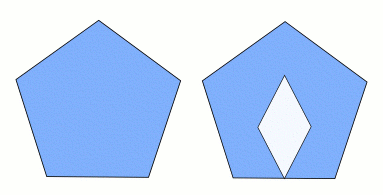Figure 2: The shape on the left is a polygon, but the one on the right is not, because it has a 'hole'.

A polygon is called regular if all of its sides are the same length, and all the angles between them are the same; the triangle and square in figure 1 and the pentagon in figure 2 are regular.

A polyhedron is what you get when you move one dimension up. It is a closed, solid object whose surface is made up of a number of polygonal faces. We call the sides of these faces edges — two faces meet along each one of these edges. We call the corners of the faces vertices, so that any vertex lies on at least three different faces. To illustrate this, here are two examples of well-known polyhedra.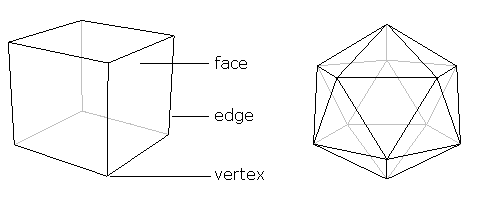Figure 3: The familiar cube on the left and the icosahedron on the right. A polyhedron consists of polygonal faces, their sides are known as edges, and the corners as vertices.

A polyhedron consists of just one piece. It cannot, for example, be made up of two (or more) basically separate parts joined by only an edge or a vertex. This means that neither of the following objects is a true polyhedron.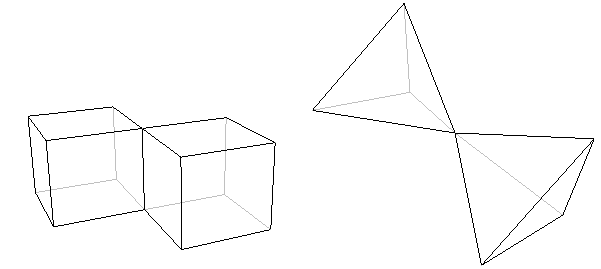Figure 4: These objects are not polyhedra because they are made up of two separate parts meeting only in an edge (on the left) or a vertex (on the right).

### What does the formula tell us?

We're now ready to see what Euler's formula tells us about polyhedra. Look at a polyhedron, for example the cube or the icosahedron above, count the number of vertices it has, and call this number V. The cube, for example, has 8 vertices, so V = 8. Next, count the number of edges the polyhedron has, and call this number E. The cube has 12 edges, so in the case of the cube E = 12. Finally, count the number of faces and call it F. In the case of the cube, F = 6. Now Euler's formula tells us that

V - E + F = 2;

or, in words: the number of vertices, minus the number of edges, plus the number of faces, is equal to two.

In the case of the cube, we've already seen that V = 8, E = 12 and F = 6. So,

V - E + F = 8 - 12 + 6 = 14 - 12 = 2

which is what Euler's formula tells us it should be. If we now look at the icosahedron, we find that V = 12, E = 30 and F = 20. Now,

V - E + F = 12 - 30 + 20 = 32 - 30 = 2,

as we expected.

Euler's formula is true for the cube and the icosahedron. It turns out, rather beautifully, that it is true for pretty much every polyhedron. The only polyhedra for which it doesn't work are those that have holes running through them like the one shown in the figure below.Figure 5: This polyhedron has a hole running through it. Euler's formula does not hold in this case.

These polyhedra are called non-simple, in contrast to the ones that don't have holes, which are called simple. Non-simple polyhedra might not be the first to spring to mind, but there are many of them out there, and we can't get away from the fact that Euler's Formula doesn't work for any of them. However, even this awkward fact has become part of a whole new theory about space and shape.

### The power of Euler's formula

Whenever mathematicians hit on an invariant feature, a property that is true for a whole class of objects, they know that they're onto something good. They use it to investigate what properties an individual object can have and to identify properties that all of them must have. Euler's formula can tell us, for example, that there is no simple polyhedron with exactly seven edges. You don't have to sit down with cardboard, scissors and glue to find this out — the formula is all you need. The argument showing that there is no seven-edged polyhedron is quite simple, so have a look at it if you're interested.

Using Euler's formula in a similar way we can discover that there is no simple polyhedron with ten faces and seventeen vertices. The prism shown below, which has an octagon as its base, does have ten faces, but the number of vertices here is sixteen. The pyramid, which has a 9-sided base, also has ten faces, but has ten vertices. But Euler's formula tells us that no simple polyhedron has exactly ten faces and seventeen vertices.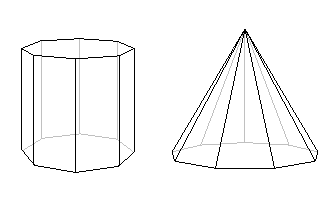Figure 6: Both these polyhedra have ten faces, but neither has seventeen vertices.

It's considerations like these that lead us to what's probably the most beautiful discovery of all. It involves the Platonic Solids, a well-known class of polyhedra named after the ancient Greek philosopher Plato, in whose writings they first appeared.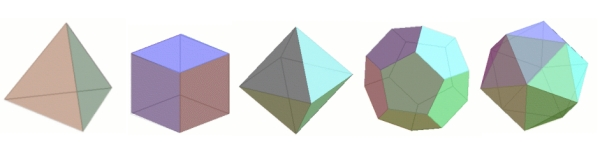Figure 7: The Platonic solids. From left to right we have the tetrahedon with four faces, the cube with six faces, the octahedron with eight faces, the dodecahedron with twelve faces, and the icosahedron with twenty faces.

Although their symmetric elegance is immediately apparent when you look at the examples above, it's not actually that easy to pin it down in words. It turns out that it is described by two features. The first is that Platonic solids have no spikes or dips in them, so their shape is nice and rounded. In other words, this means that whenever you choose two points in a Platonic solid and draw a straight line between them, this piece of straight line will be completely contained within the solid — a Platonic solid is what is called convex. The second feature, called regularity, is that all the solid's faces are regular polygons with exactly the same number of sides, and that the same number of edges come out of each vertex of the solid.

The cube is regular, since all its faces are squares and exactly three edges come out of each vertex. You can verify for yourself that the tetrahedron, the octahedron, the icosahedron and the dodecahedron are also regular.

Now, you might wonder how many different Platonic Solids there are. Ever since the discovery of the cube and tetrahedron, mathematicians were so attracted by the elegance and symmetry of the Platonic Solids that they searched for more, and attempted to list all of them. This is where Euler's formula comes in. You can use it to find all the possibilities for the numbers of faces, edges and vertices of a regular polyhedron.What you will discover is that there are in fact only five different regular convex polyhedra! This is very surprising; after all, there is no limit to the number of different regular polygons, so why should we expect a limit here? The five Platonic Solids are the tetrahedron, the cube, the octahedron, the icosahedron and the dodecahedron shown above.

### The proofRené Descartes,
(1596 - 1650)

Playing around with various simple polyhedra will show you that Euler's formula always holds true. But if you're a mathematician, this isn't enough. You'll want a proof, a water-tight logical argument that shows you that it really works for all polyhedra, including the ones you'll never have the time to check.Despite the formula's name, it wasn't in fact Euler who came up with the first complete proof. Its history is complex, spanning 200 years and involving some of the greatest names in maths, including René Descartes (1596 - 1650), Euler himself, Adrien-Marie Legendre (1752 - 1833) and Augustin-Louis Cauchy (1789 - 1857).Augustin-Louis Cauchy, (1789 - 1857)

It's interesting to note that all these mathematicians used very different approaches to prove the formula, each striking in its ingenuity and insight. It's Cauchy's proof, though, that I'd like to give you a flavour of here. His method consists of several stages and steps. The first stage involves constructing what is called a network.

### Forming a network

Imagine that you're holding your polyhedron with one face pointing upward. Now imagine "removing" just this face, leaving the edges and vertices around it behind, so that you have an open "box". Next imagine that you can hold onto the box and pull the edges of the missing face away from one another. If you pull them far enough the box will flatten out, and become a network of points and lines in the flat plane. The series of diagrams below illustrates this process as applied to a cube.Figure 8: Turning the cube into a network.

As you can see from the diagram above, each face of the polyhedron becomes an area of the network surrounded by edges, and this is what we'll call a face of the network. These are the interior faces of the network. There is also an exterior face consisting of the area outside the network; this corresponds to the face we removed from the polyhedron. So the network has vertices, straight edges and polygonal faces.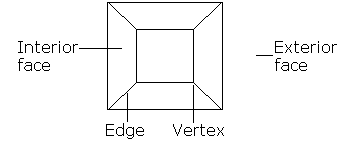Figure 9: The network has faces, edges and vertices.

When forming the network you neither added nor removed any vertices, so the network has the same number of vertices as the polyhedron — V. The network also has the same number of edges — E — as the polyhedron. Now for the faces; all the faces of the polyhedron, except the "missing" one, appear "inside" the network. The missing face has become the exterior face which stretches away all round the network. So, including the exterior face, the network has F faces. Thus, you can use the network, rather than the polyhedron itself, to find the value of V - E + F. We'll now go on to transform our network to make this value easier to calculate.

### Transforming the Network

There are three types of operation which we can perform upon our network. We'll introduce three steps involving these.

Step 1 We start by looking at the polygonal faces of the network and ask: is there a face with more than three sides? If there is, we draw a diagonal as shown in the diagram below, splitting the face into two smaller faces.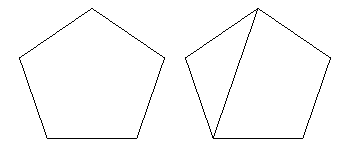Figure 10: Dividing faces.

We repeat this with our chosen face until the face has been broken up into triangles.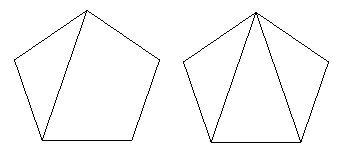Figure 11: In the end we are left with triangular faces.

If there is a further face with more than three sides, we use Step 1 on that face until it too has been broken up into triangular faces. In this way, we can break every face up into triangular faces, and we get a new network, all of whose faces are triangular. We illustrate this process by showing how we would transform the network we made from a cube.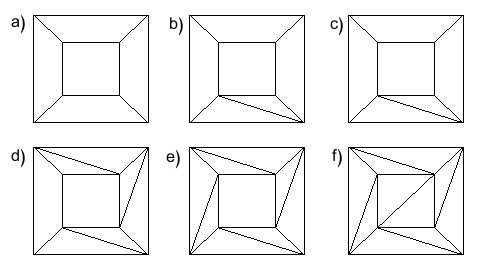Figure 12: This is what happens to the cube's network as we repeatedly perform Step 1.

We go back to Step 1, and look at the network we get after performing Step 1 just once. Now, by drawing a diagonal we added one edge. Our original face has become two faces, so we have added one to the number of faces. We haven't changed the number of vertices. The network now has V vertices, E + 1 edges and F + 1 faces. So how has V - E + F changed after we performed Step 1 once? Using what we know about the changes in V, E and F we can see that V - E + F has become V - (E + 1) + (F + 1). Now we have

V - (E + 1) + (F + 1) = V - E - 1 + F + 1 = V - E + F.

So V - E + F has not changed after Step 1! Because each use of Step 1 leaves V - E +  F unchanged, it is still unchanged when we reach our new network made up entirely of triangles! The effect on V - E + F as we transform the network made from the cube is shown in the table below.

 Round V E F V - E + F (a) 8 12 6 2 (b) 8 13 7 2 (c) 8 14 8 2 (d) 8 15 9 2 (e) 8 16 10 2 (f) 8 16 11 2

We now introduce Steps 2 and 3. They will remove faces from around the outside of the network, reducing the number of faces step by step. Once we begin to do this the network probably won't represent a polyhedron anymore, but the important property of the network is retained.

Step 2 We check whether the network has a face which shares only one edge with the exterior face. If it does, we remove this face by removing the one shared edge. The area which had been covered by our chosen face becomes part of the exterior face, and the network has a new boundary. This is illustrated by the diagram below for the network made from the cube.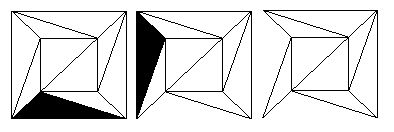Figure 13: Removing faces with one external edge.

Now, we will take V, E and F to be the numbers of vertices, edges and faces the network made up of triangular faces had before we performed Step 2. We now look at how the number V - E + F has changed after we perform Step 2 once. We have removed one edge, so our new network has E - 1 edges. We have not touched the vertices at all, so we still have V vertices. The face we used for Step 2 was merged with the exterior face, so we now have F - 1 faces. So V - E + F has become V - (E - 1) + (F - 1) and

V - (E - 1) + (F - 1) = V - E + 1 + F - 1 = V - E + F.

So once again V - E +  F has not changed.

Step 3 We check whether our network has a face which shares two edges with the exterior face. If it does, we remove this face by removing both these shared edges and their shared vertex, so that again the area belonging to our chosen face becomes part of the exterior face. This is illustrated below in the case of the network made from the cube, as it is after performing Step 2 twice.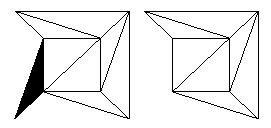Figure 14: Removing faces with two external edges.

As we did before we now take V, E and F to be the numbers of vertices, edges and faces of the network we're starting with. Now how has the number V - E + F been affected by Step 3? We have removed one vertex — the one between the two edges — so there are now V - 1 vertices. We have removed two edges, so there are now E - 2 edges. Finally, our chosen face has merged with the exterior face, so we now have F - 1 faces. So V - E + F has become (V - 1) - (E - 2) - (F - 1) and

(V - 1) - (E -2) + (F - 1) = V - 1 - E + 2 + F - 1 = V - E + F.

So once more V - E + F has not changed.

The secret of the proof lies in performing a sequence of Steps 2 and 3 to obtain a very simple network. Recall that we had repeatedly used Step 1 to produce a network with only triangular faces. This network will definitely have a face which shares exactly one edge with the exterior face, so we take this face and perform Step 2. We can perform Step 2 on several faces, one at a time, until a face sharing two edges with the exterior face appears. We can then perform Step 3 using this face. We carry on performing Steps 2 and 3, and keep removing faces in this way.

There are two important rules to follow when doing this. Firstly, we must always perform Step 3 when it's possible to do so; if there's a choice between Step 2 and Step 3 we must always choose Step 3. If we do not, the network may break up into separate pieces. Secondly, we must only remove faces one at a time. If we don't we may end up with edges sticking out on their own into the exterior face, and we'll no longer have a proper network. To illustrate the process, we'll perform several steps on the cube network, continuing from where we left it in the last diagram.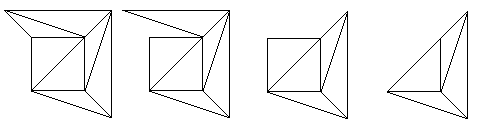Figure 15: Applying our algorithm to the network of the cube.

Now we can ask ourselves one or two questions. Does this process of removing faces ever stop, and, if it does, what are we left with? A little consideration will show you that it must stop — there are only finitely many faces and edges we can remove — and that when it does, we are left with a single triangle. You can see some diagrams describing the whole process for the network formed from a dodecahedron (recall that this was one of the Platonic solids introduced earlier).

Now look at the numbers of vertices, edges and faces present in our final network — the single triangle. We have V=3, E=3, and F=2 — we must still include the exterior face. Now

V - E + F = 3 - 3 + 2 = 2.

Throughout the whole process, starting with the complete polyhedron and ending with a triangle, the value of V - E + F has not changed. So if V - E + F = 2 for the final network, we must also have V - E + F = 2 for the polyhedron itself! The proof is complete!

• 0

f+v=e+2

• 0
What are you looking for?